# What Affects Probability? (5 Things You Need To Know)

Probability depends on the event we wish to observe and the conditions of the scenario.  It may also depend on related events and their probabilities.

So, what affects probability?  Probability is the number of desired outcomes divided by the total outcomes. Five factors that affect probability are unions, intersections, conditionals, independence of events, and mutual exclusivity of events. More information about a scenario can change the probability we assign to an event.

Of course, we still need to figure out (or be told) that events are independent or mutually exclusive.

In this article, we’ll talk about what affects probability.  We’ll also look at some examples to see how these 5 factors can change the numbers we get when calculating probability.

Let’s get started.

## What Affects Probability?

Remember that the probability of an event A is given by:

• P(A) = D/T

where D is the number of desired outcomes and T is the total number of possible outcomes.

For example, if A is the event that you roll a 1 on a fair 6-sided die, then P(A) = 1/6, since there is 1 desired outcome (rolling a 1) and 6 possible outcomes (rolling 1, 2, 3, 4, 5, or 6).

Here are 5 things that affect probability (or tell you something about its value):

• Unions – the notation AuB means “A union B”, and P(AuB) is the probability that A or B (or both) occur.
• Intersections – the notation AnB means “A intersection B”, and P(AnB) is the probability that A and B both occur.  This is also called the joint probability of A and B.
• Conditionals – the notation A|B means “A given B”, and P(A|B) is the probability that A occurs, given that B has already occurred.
• Independence – A and B are independent if the occurrence of one does not affect the occurrence (probability) of the other.  For example, the outcome of the first roll of a 6-sided die does not affect the outcome of the second roll, so the two rolls are independent.
• Mutual Exclusivity – A and B are mutually exclusive if they cannot both happen (for example, it cannot be 50 degrees Fahrenheit and 90 degrees Fahrenheit at the same time).  For example, we cannot flip both heads and tails on a coin in the same flip, so the outcomes are mutually exclusive.

Note that AuB “contains” AnB, meaning P(AuB) >= P(AnB) for any events A and B.  The only time P(AuB) and P(AnB) are equal is when A and B cannot occur independently of one another.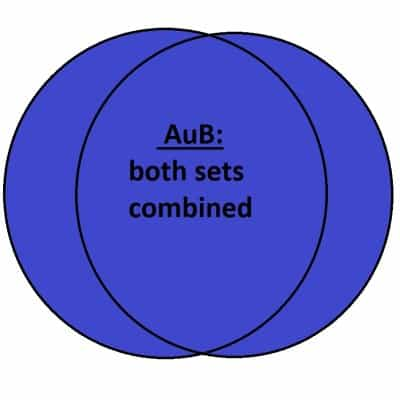Here we see AuB, the union of A and B. Note that AuB contains all of AnB.

Here are some key equations that relate the concepts above:

• P(AuB) = P(A) + P(B) – P(AnB).  Note that this formula is equivalent to P(AuB) = P(A) + P(B) – P(A)P(B) when A and B are independent events.  Also note that this formula simplifies to P(AuB) = P(A) + P(B) when P(AnB) = 0; that is, when A and B are mutually exclusive or disjoint (i.e. they cannot occur together).
• P(AnB) = P(A)*P(B) when A and B are independent events (that is, when the occurrence of one does not affect occurrence of the other).  If A and B are both independent and mutually exclusive, then P(A)*P(B) = 0, meaning one or both probabilities are zero.
• P(A|B) = P(AnB)/P(B).  Note that this formula simplifies to P(A|B) = P(A) if A and B are independent events.  Also, note that P(A|B) = 0 if A and B are mutually exclusive events.

Let’s look at some examples to see how the numbers work in various scenarios.

### Example 1: Probability Of A Union With Mutually Exclusive Events

Let’s say that we are going to roll a six-sided die.  We want to find the probability of rolling a 1 or a 2.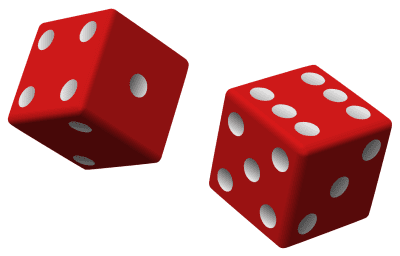We can use a union to find the probability of one event or another occurring on a die roll.

So our events are:

• A = the event that the die roll comes up at “1”.
• B = the event that the die roll comes up at “2”.

and our probabilities are:

• P(A) = 1/6 (there is 1 way to roll a 1, and 6 possible outcomes)
• P(B) = 1/6 (there is 1 way to roll a 2, and 6 possible outcomes)
• P(AnB) = 0 (there is no way to roll a 1 and a 2 on the same roll; that is, A and B are mutually exclusive)

Using the formula for a union (rolling a 1 or a 2), we get:

• P(AuB) = P(A) + P(B) – P(AnB)
• P(AuB) = 1/6 + 1/6 – 0
• P(AuB) = 2/6
• P(AuB) = 1/3

### Example 2: Probability Of A Union With Independent Events

Let’s say that we are going to roll a six-sided die and flip a coin.  We want to find the probability of rolling a 1 on the die or flipping heads on the coin.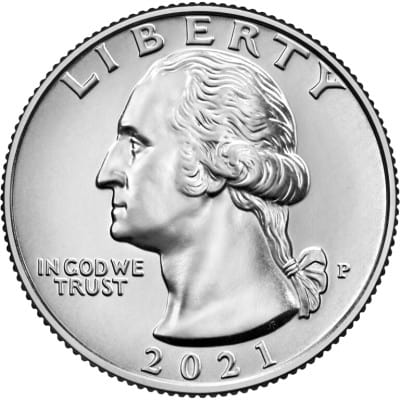We can also include a coin flip in our probability calculations. Assuming a fair coin, the probabilities are 50% (0.5) for both heads and tails.

So our events are:

• A = the event that the die roll comes up at “1”.
• B = the event that the coin toss comes up as “heads”.

and our probabilities are:

• P(A) = 1/6 (there is 1 way to roll a 1, and 6 possible outcomes)
• P(B) = 1/2 (there is 1 way to flip heads, and 2 possible outcomes)
• P(AnB) = (1/6)*(1/2) = 1/12 (the die roll and coin flip do not affect each other, meaning they are independent events, so the joint probability is the product of the probabilities)

Using the formula for a union (rolling a 1 or flipping heads), we get:

• P(AuB) = P(A) + P(B) – P(AnB)
• P(AuB) = 1/6 + 1/2 – 1/12
• P(AuB) = 2/12 + 6/12 – 1/12
• P(AuB) = (2 + 6 – 1)/12
• P(AuB) = 7/12

### Example 3: Probability Of An Intersection With Independent Events

Let’s say that we are going to roll a six-sided die and flip a coin.  We want to find the probability of rolling a 1 on the die and flipping heads on the coin.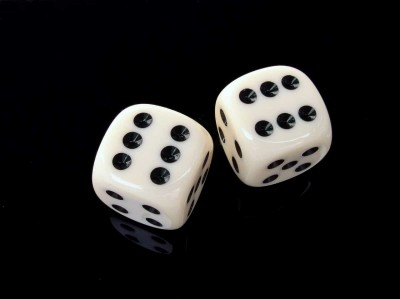We can combine rolling dice and flipping coins to find the probabilities for intersections of events.

So our events are:

• A = the event that the die roll comes up at “1”.
• B = the event that the coin toss comes up as “heads”.

and our probabilities are:

• P(A) = 1/6 (there is 1 way to roll a 1, and 6 possible outcomes)
• P(B) = 1/2 (there is 1 way to flip heads, and 2 possible outcomes)
• P(AnB) = (1/6)*(1/2) = 1/12 (the die roll and coin flip do not affect each other, meaning they are independent events, so the joint probability is the product of the probabilities)

### Example 4: Conditional Probability With Independent Events

Let’s say that we are going to roll two six-sided dice and sum their faces.  After rolling, the first die on the table reads 3.

The second die falls on the floor and rolls under the refrigerator (this always happens to me!)  Before we move the heavy refrigerator and read the face on the 2nd die: what is the probability that the sum on both faces is at least 7?

So our events are:

• A = the event that the first die roll comes up “3”
• B = the event that the sum of the faces of the two dice is at least 6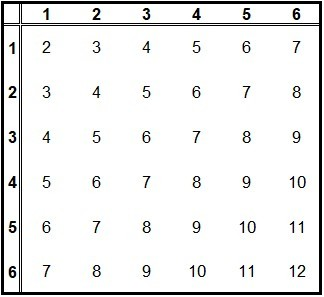This table shows the possible combinations for a roll of two dice. There are 10 sums less than 6, so there are 36 – 10 = 26 sums that are at least 6. How many ways can you get a total of at least 6 if one die already shows a 3?

So we are looking for a conditional probability, P(B|A) – the probability that the sum of the dice comes out to at least 6, given that the first one comes out to a 3.

Our probabilities are:

• P(A) = 1/6 (there is 1 way to roll a 3, and 6 possible outcomes)
• P(AnB) = (1/6)*(4/6) = 4/36 = 1/9

Using the formula for conditional probability, we get:

• P(B|A) = P(AnB)/P(A)
• P(B|A) = (1/9)/(1/6)
• P(B|A) = 2/3

This makes sense, since a roll of 3, 4, 5, or 6 on the 2nd die will result in a total of at least 6.  That means 4 of the 6 possible outcomes (2/3) will give us a sum of 6 or more.

### Example 5: Probability Of Both Union & Intersection

Let’s say that we are going to roll two six-sided dice to find their product, and we will also flip a coin.  We want to find the probability of rolling a product of 12 on the dice or flipping tails on the coin.

So our events are:

• A = the event that the dice roll comes out to a product of “12”
• B = the event that the coin toss comes up as “tails”.

and our probabilities are:

• P(A) = 4/36 = 1/9 (there are 4 ways to roll a product of 12: 2*6, 3*4, 4*3, and 6*2, with 36 possible outcomes)
• P(B) = 1/2 (there is 1 way to flip tails, and 2 possible outcomes)
• P(AnB) = (1/9)*(1/2) = 1/18 (the die rolls and coin flip do not affect each other, meaning they are independent events, so the joint probability is the product of the probabilities)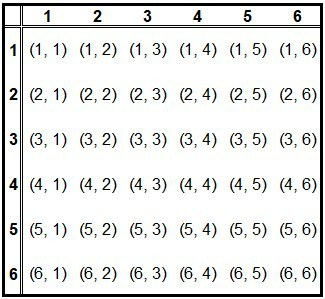This table shows the possible combinations for a roll of two dice. The only ways to get a product of 12 is (2, 6), (3, 4), (4, 3), and (6, 2).

Using the formula for a union (rolling a product of 12 or flipping tails), we get:

• P(AuB) = P(A) + P(B) – P(AnB)
• P(AuB) = 1/9 + 1/2 – 1/18
• P(AuB) = 2/18 + 9/18 – 1/18
• P(AuB) = (2 + 9 – 1)/18
• P(AuB) = 10/18
• P(AuB) = 5/9

## Conclusion

Now you know what affects probability and how to make calculations for various scenarios, including unions, intersections, and conditionals, as well as independence and mutual exclusivity.

You can learn about the Monty Hall Problem (an example of conditional probability) here.